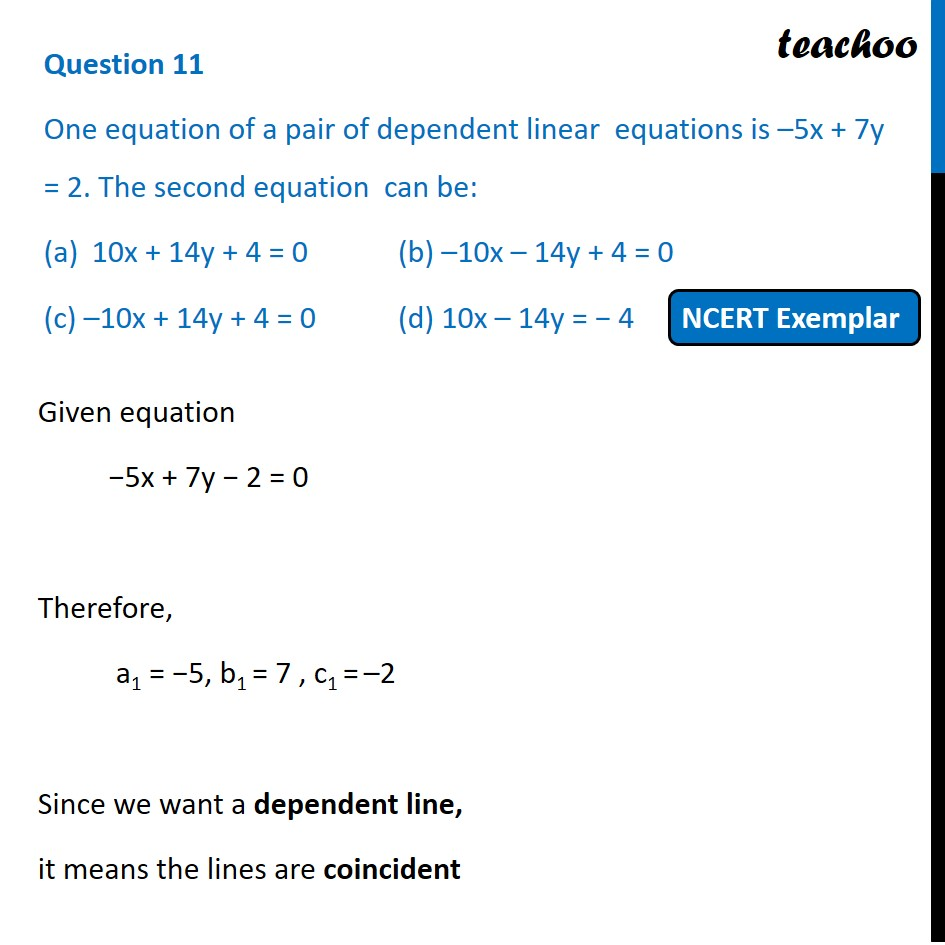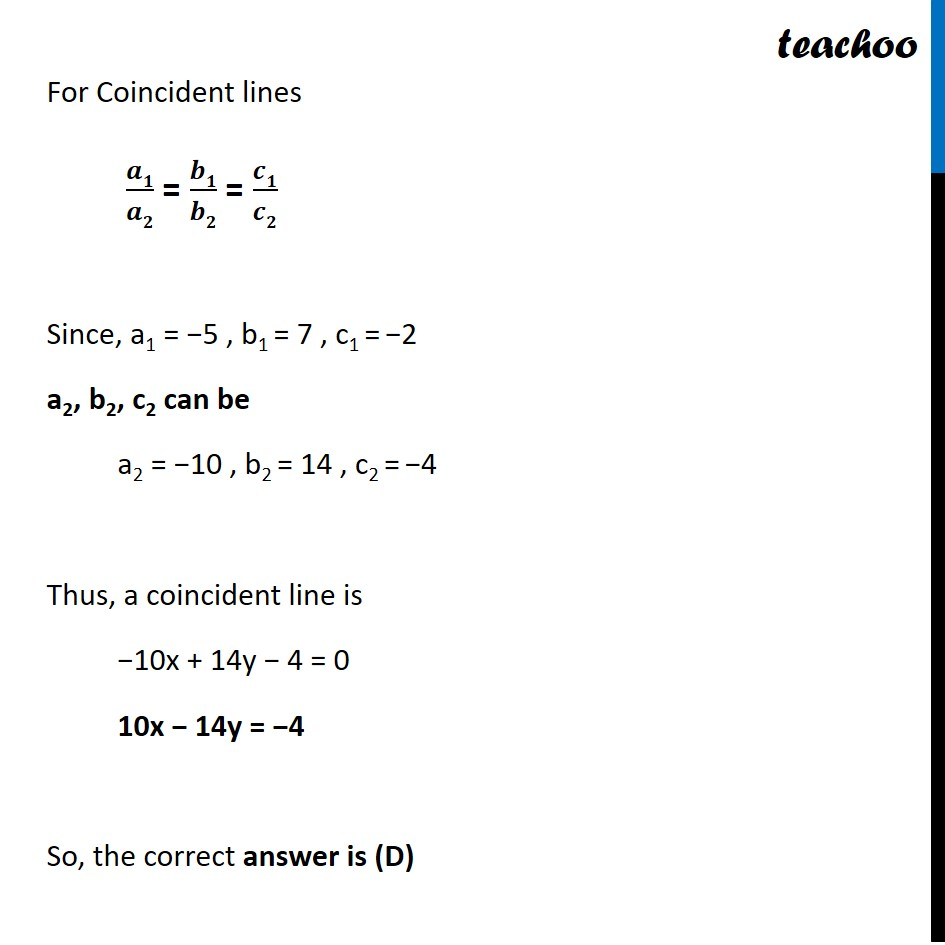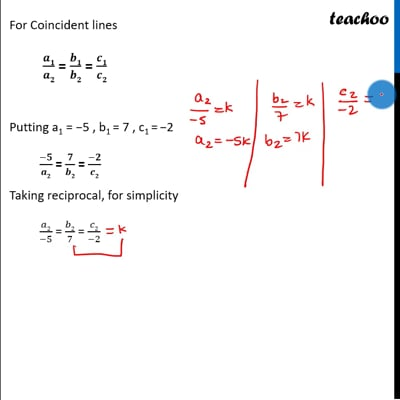MCQs from NCERT Exemplar

Chapter 3 Class 10 Pair of Linear Equations in Two Variables (Term 1)
Serial order wise

## (c) –10x + 14y + 4 = 0   (d) 10x – 14y = − 4This video is only available for Teachoo black users

### Transcript

Question 11 One equation of a pair of dependent linear equations is –5x + 7y = 2. The second equation can be: 10x + 14y + 4 = 0 (b) –10x – 14y + 4 = 0 (c) –10x + 14y + 4 = 0 (d) 10x – 14y = − 4 Given equation −5x + 7y − 2 = 0 Therefore, a1 = −5, b1 = 7 , c1 = –2 Since we want a dependent line, it means the lines are coincident For Coincident lines 𝒂𝟏/𝒂𝟐 = 𝒃𝟏/𝒃𝟐 = 𝒄𝟏/𝒄𝟐 Since, a1 = −5 , b1 = 7 , c1 = −2 a2, b2, c2 can be a2 = −10 , b2 = 14 , c2 = −4 Thus, a coincident line is −10x + 14y − 4 = 0 10x − 14y = −4 So, the correct answer is (D)